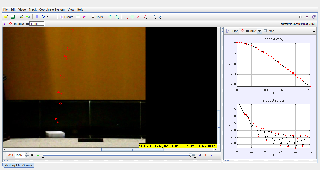### Software Requirements

SoftwareRequirements

 Android iOS Windows MacOS with best with no no need Java need Java support full-screen? no no Yes Yes cannot work on no mobile browser  understand Java.... no mobile browser  understand Java.... install Tracker from http://physlets.org/tracker/ install Tracker from http://physlets.org/tracker/

### Theory

the equation for the drag force on the square paper is may be assumed to be is the form of $$F = \frac{1}{2} \rho v^{2} C_{D} A$$

where F is the aerodynamic drag force

$$\rho$$ is the is the density of the fluid

$$v$$ is the speed of the object relative to the fluid

$$A$$ is the cross sectional area

$$C_{D}$$  is the drag coefficient – a dimensionless number.

### Data

 Area, A/ $$cm^{2}$$ 289 249 190 169 130 88 Terminal Velocity, v/ $$cms^{-1}$$ 1.66 1.7 2.02 2.12 2.31 2.66

### Version

http://weelookang.blogspot.sg/2016/08/sypt2016-workshop-materials.html

### end faq

Rating 5.00 (1 Vote)